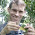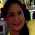## Friday, March 16, 2012

### Phylogenetic signal with K and λ

A recent Google search string that led a reader to my blog was the following:

different phylogenetic signal with K vs lambda.

Phylogenetic signal is generally recognized to be the tendency of related species to resemble one another; and Blomberg et al.'s (2003) K and Pagel's (1999) λ are two quantitative measures of this pattern. The metrics are quite different from one another. λ is a scaling parameter for the correlations between species, relative to the correlation expected under Brownian evolution. K is a scaled ratio of the variance among species over the contrasts variance (the latter of which will be low if phylogenetic signal is high). λ has a nice natural scale between zero (no correlation between species) and 1.0 (correlation between species equal to the Brownian expectation). λ itself is not a correlation, but a scaling factor for a correlation, so λ>1.0 is theoretically possible. However, depending on the structure of the tree, λ>>1.0 is usually not defined. K, a variance ratio, is rescaled by dividing by the Brownian motion expectation. This gives it the property of having an expected value of 1.0 under Brownian evolution, but K for empirical and simulated datasets can sometimes be >>1.0. Since they measure the qualitative tendency towards similarity of relatives in entirely different ways, we have no real expectation that they will be numerically equivalent - except (by design) under Brownian motion evolution.

So, accepting that λ & K are different measures of phylogenetic signal, we might reasonably ask - do statistical tests based on each measure tend to find significant phylogenetic signal for the same datasets? One way to crudely test this would be simulate datasets for various generating λ and then ask if significant tests based on λ and K tend to be associated.

require(phytools); require(geiger)
nrep<-1000
P.K<-P.l<-l<-vector()
for(i in 1:nrep){
tree<-pbtree(n=50)
l[i]<-runif(n=1)
x<-fastBM(lambdaTree(tree,l[i]))
K<-phylosig(tree,x,test=T)
lambda<-phylosig(tree,x,method="lambda",test=T)
P.K[i]<-K\$P
P.l[i]<-lambda\$P
}

Compute the fraction of significant tests using K & λ, and the fraction expected to be the same (either both significant or both non-significant) if they are independent (this we compute as the product of the preceding fractions plus the product of 1 minus each fraction):

> pK<-mean(P.K<=0.05); pK
 0.417
> pL<-mean(P.l<=0.05); pL
 0.514
> pK*pL+(1-pK)*(1-pL)
 0.497676

Now, the fraction of times that tests on λ & K yield the same result (i.e., either both significant or both non-significant):

> mean(((P.K<=0.05)==(P.l<=0.05)))
 0.765

Obviously, there is some relation between the tests of the two metrics, but it is not extremely strong. Let's also ask if the average generating value of λ is higher when either or both λ and K produce a significant result:

> # first lambda
> mean(l[P.l<=0.05]) # lambda significant
 0.6671113
> mean(l[P.l>0.05]) # not significant
 0.2884
> # then K
> mean(l[P.K<=0.05]) # K significant
 0.6842788
> mean(l[P.K>0.05]) # not significant
 0.339131
> # then P & lambda
> mean(l[(P.K<=0.05)*(P.l<=0.05)==1]) # lambda & K significant
 0.7352098
> mean(l[(P.K<=0.05)*(P.l<=0.05)==0]) # neither significant
 0.3484733

1.Hi,

I used this function to estimate both lambda and K for a data set of 95 species. The estimated K was 0.54 (p=0.013) and lambda was 0.98 (p=0.001). Given how I've understood lambda and Blomberg's own explanation if K:

"A K less than one implies that relatives resemble each other less than expected under Brownian motion evolution along the candidate tree. This could be caused by either departure from Brownian motion evolution, such as adaptive evolution that is uncorrelated with the phylogeny (i.e., homoplasy), or ‘‘measurement error’’ in the broad sense."

There must be something not quite right here? K tells me there is weak phylogenetic dependence, while lambda returns a value that indicates near perfect phylogentic dependence.

Any tips?

1.K & λ are not the same thing. λ measures the similarity of the covariances among species to the covariances expected under Brownian motion; whereas K might be more usefully thought of as a measure of the partitioning of variance. If K>1 then variance tends to be among clades; while if K<1 then variance is within clades (with BM as reference). The variance on K for a given process is quite large. I suspect if you did simulation you might find that K=0.54 (particularly for a relatively small tree) was not different from BM. E.g.:

> tree<-pbtree(n=50)
> X<-fastBM(tree,nsim=1000)
> K<-apply(X,2,phylosig,tree=tree)
> quantile(K,c(0.05,0.95))
5% 95%
0.4203233 2.0659019

- Liam

2.Oh, my. That's a big confidence interval.

Thanks for clearing this up...

Jostein

2.Hi Liam,

as far as I understand, the p value for lambda indicates if my value for lambda is significantly different from zero. Is there a way of testing in phytools whether lambda is also significantly different from 1?

Cheers,
Anna

1.There is Anna - you could just use a likelihood ratio test against a Brownian model. If you don't know how to do this, you could email me directly or the R-sig-phylo list. I have trouble keeping up with comments on my blog - particularly on old posts. - Liam

3.Hi

I have problems with consentrait. My tree labels not match with data table.

\$tree_not_data

Can you help me?

Tanks

Note: due to the very large amount of spam, all comments are now automatically submitted for moderation.